# GMAT Quant: Mathematical Grouping Symbols

Many folks remember Order of Operations by means of the mnemonic PEMDAS.  Just for review, PEMDAS is a quick way to remember the following order of mathematical prioritization:

Priority #1: P = Parentheses

Priority #2: E = Exponents

Priority #3: MD = Multiplication & Division

Priority #4: AS = Addition and Subtraction

A few things to notice about this.  First of all, within the parentheses, you can have any combination of symbols, so the order of mathematical prioritization would be repeated inside the parentheses (exponents inside the parentheses, then multiplication & division inside the parenthesis, then addition & subtraction inside the parenthesis); once the entire contents of the parentheses have been simplified to a single number, the entire parenthetical expression, parentheses and all, get replaced by this single number, and then outside-the-parentheses operations can proceed according to their prioritization levels.   If we have nested parentheses, then this process is extended, with the innermost parentheses getting the highest prioritization.

Notice also: multiplication and division are at the same level of mathematical prioritization.  Some mathematicians would say that, really, division doesn’t exist: when we think we are “dividing by 7”, we are really multiplying by 1/7.  To some extent, that’s a semantic distinction, but it does underscore the profound relationship between multiplication and division.  As long as we don’t change which factors are multiplied and by which we divide, we can swap around the order in any way we like.  For example: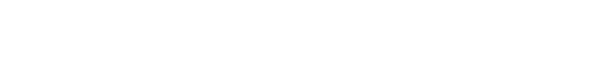Each of those is 100% correct, and they are all perfectly valid reorganizations of each other.   One extremely practical implication of this concerns how you might deal with, for example, the following fraction: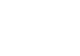Suppose you need to simplify this in a GMAT problem.  Many people, doing math on automatic pilot, will begin by multiplying 48 times 91. Since you get no calculator on the GMAT Quant section, this beginning is just about the most boneheaded move one could possibly make.   Starting with that multiplication is the mathematical equivalent of driving from NYC to Boston by route of Albuquerque, NM.  Now, Albuquerque, NM is an truly lovely town in its own right, but if you start in NYC and just want to get to Boston, Albuquerque is more than a little bit out of the way!  If you multiply 48 time 91, to get whatever four-digit monstrosity you get, you have gone way out of your way and you have made the problem 15 times harder than it has to be.  Instead, CANCEL BEFORE YOU MULTIPLY.  For exampleON the GMAT, there is almost NEVER a reason you will have to do any kind of significant arithmetic with anything larger than a two-digit number.  If you wind up with a four-digit number that you have to multiply or divide, know that you inadvertently have made a choice somewhere along the way to make the problem much harder than it had to be.

## More on canceling

The foregoing section address the aggressive and productive cancelling you are 100% allowed to employ if everything is either multiplied or divided — if there’s no addition or subtraction mucking up the works.  What kind of cancelling is allowed if there is addition or subtraction involved?  Consider the following question.  (This is not a standard GMAT Quant question format: this “multiple answer” format never appears on the GMAT, but does appear on the GRE).In my teaching career, I have seen analogs of each of the nine of these appear, at various times, in a student’s work as something that the student believes is a valid form of cancelling.  In fact, only (H) is correct, and the other eight are hideously incorrect, yet folks arrive at many of those others time and time again.   What’s going on here?

You see, that long horizontal “fraction bar” that divide the numerator from the denominator —- that is something mathematically know as a grouping symbol.  Parentheses & brackets are the most common grouping symbol, but the horizontal fraction bar is another.  For example, suppose we wanted to write this original fraction in plaintext.  Many Magoosh users, when they send us support emails, have to write complicated fractions in plaintext.  First of all, here is the wrong way to do it.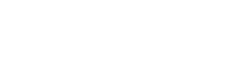That is an unholy abomination.  Technically,  the right side of that equation say: take 12x by itself, add it to 36 divided by 4x, and then add that to 6.  That’s what the right says, which is completely different from what the left side says.  To say that these two are equal is a mathematical obscenity!  And yet, many students rewrite the left side as the right side, without any idea of the problem.

The long horizontal fraction bar of a properly written fraction, a fraction with the numerator vertically above the denominator — that fraction bar is a grouping symbol.  We can’t express that grouping symbol in plaintext, and the simple slash, /, is NOT a grouping symbol.   That’s part of the problem — the left side, the true fraction, has a bonafide grouping symbol, and the red expression on the right is devoid of one.  Therefore, we need to substitute the only grouping symbol allowed in plaintext: parentheses.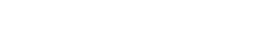Now, that’s 100% mathematically correct.  First of all, this is important for any to appreciate who has to write about a fraction as part of asking a question in an email, in a forum post, etc.   BUT notice: this is not merely a matter of persnickety bookkeeping.  In fact, this difference makes explicit a profound mathematical issue.

Think about the cancelling question above.  If we rewrite the vertical fraction as a plaintext fraction, we have to include the parentheses, and that makes explicitly visible the way elements are groups.  Order of operations tells us that operations inside parentheses must take priority, so we can’t cancel, say, the 12x with the 4x, because they are included in separate calculations.

Rewriting the fraction in the form involving the parentheses makes clearer how order of operations comes into play, but of course, the way we write the fraction doesn’t change what legitimately can be cancelled.  No matter what form the fraction is in, we can’t cancel that 12x with that 4x, nor that 36 with that 6.  Technically, order of operations is not PEMDAS but GEMDAS, where the G = grouping symbols, which includes not only parentheses and brackets but also structures such as horizontal fraction bars.   All of these things take precedence over the other arithmetic operations.

Now, having said that, it may seem to some readers that no cancelling is ever possible if there are algebraic expressions in the numerator and denominator.  While the possibilities for cancelling are far fewer than the mathematically naïve would suppose, there is one possible route.  Suppose we can factor out something from both the numerator and denominator: for example, with this fraction —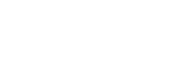Now, as discussed in the first section, we can rearrange multiplication and division in any order, so we can cancel those factored-out numerical factors.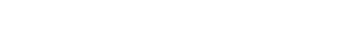This is precisely how we arrived at answer H above.

## More on grouping symbols

Another grouping symbol is the “exponent slot” (that’s not an official term — I just don’t know what else to call it!!)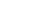The “x+3” is “grouped” in the exponent.  Writing the ordinary superscript exponents makes this grouping clear.   When we have to write something like this in plaintext, as with fractions, we lose the original grouping symbol.  Many students make atrocious mistakes when writing something like this in plaintext.  For example: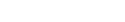This too is an unholy abomination.   Literally, the right side now says: raise 2 to the power of x, and when you are done with that, add 3 to the result.  That’s what the right side says, which of course, is completely different from what the left side says.  As in the fraction case, we have lost a grouping symbol, and we need to replace it with the only grouping symbol allowed in plaintext: parentheses.  BUT, we must be very careful where we put the parentheses.  For example: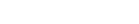This is an unholy abomination in parentheses.  This change is like choosing a more attractive font with which to write an obscene word: it’s a cosmetic difference that doesn’t address the core issue.   We only fix the problem if we precisely replace one grouping symbol with another grouping symbol: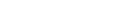This is now 100% correct.  Again, more than a matter of simple notation, these difference demonstrate something quite profound about the underlying mathematical relationships. The plaintext format of emails and forums is annoying in some ways, but in some ways it makes explicit some mathematical ideas of which some students might have remained unaware.

## Summary

I hope this post gave you some insight into mathematical notation and its logical consequences.  Here’s an easy question that employs some related ideas:

•